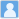• ### String length constraining from the stochastic gravitational waves background

分类： 天文学 >> 天文学 提交时间： 2023-02-19Hongguang Zhang Xilong Fan Yufeng Li Minglei Tong Hongsheng Zhang

摘要：We study the stochastic gravitational waves from string gas cosmology. With the help of the Lambert W function, we derive the exact energy density spectrum of the stochastic gravitational waves in term of tensor-to-scalar. New feathers with the spectrum are found. First, the non-Hagedorn phase can be ruled out by the current B-mode polarization in the cosmic microwave background. Second, the exact spectrum from the Hagedorn phase with a logarithmic term is shown to be unique in the measurable frequency range. Third, which is the most important, we find the string length can be constrained to be lower than 7 $\sim$ orders of that Planck scale.

同行评议状态:待评议

• ### Exploring the sky localization and early warning capabilities of third generation gravitational

分类： 天文学 >> 天文学 提交时间： 2023-02-19Yufeng Li Ik Siong Heng Man Leong Chan Chris Messenger Xilong Fan

摘要：This work characterises the sky localization and early warning performance of networks of third generation gravitational wave detectors, consisting of different combinations of detectors with either the Einstein Telescope or Cosmic Explorer configuration in sites in North America, Europe and Australia. Using a Fisher matrix method which includes the effect of earth rotation, we estimate the sky localization uncertainty for $1.4\text{M}\odot$-$1.4\text{M}\odot$ binary neutron star mergers at distances $40\text{Mpc}$, $200\text{Mpc}$, $400\text{Mpc}$, $800\text{Mpc}$, $1600\text{Mpc}$, and an assumed astrophysical population up to redshift of 2 to characterize its performance for binary neutron star observations. We find that, for binary neutron star mergers at $200\text{Mpc}$ and a network consisting of the Einstein Telescope, Cosmic Explorer and an extra Einstein Telescope-like detector in Australia(2ET1CE), the upper limit of the size of the 90% credible region for the best localized 90% signals is $0.25\text{deg}^2$. For the simulated astrophysical distribution, this upper limit is $91.79\text{deg}^2$. If the Einstein Telescope-like detector in Australia is replaced with a Cosmic Explorer-like detector(1ET2CE), for $200\text{Mpc}$ case, the upper limit is $0.18\text{deg}^2$, while for astrophysical distribution, it is $56.77\text{deg}^2$. We note that the 1ET2CE network can detect 7.2% more of the simulated astrophysical population than the 2ET1CE network. In terms of early warning performance, we find that a network of 2ET1CE and 1ET2CE networks can both provide early warnings of the order of 1 hour prior to merger with sky localization uncertainties of 30 square degrees or less. Our study concludes that the 1ET2CE network is a good compromise between binary neutron stars detection rate, sky localization and early warning capabilities.

同行评议状态:待评议

• ### Model Independent Approach of the JUNO $^8$B Solar Neutrino Program

分类： 天文学 >> 天文学 提交时间： 2023-02-19

摘要：The physics potential of detecting $^8$B solar neutrinos is exploited at the Jiangmen Underground Neutrino Observatory (JUNO), in a model independent manner by using three distinct channels of the charged-current (CC), neutral-current (NC) and elastic scattering (ES) interactions. Due to the largest-ever mass of $^{13}$C nuclei in the liquid-scintillator detectors and the potential low background level, $^8$B solar neutrinos would be observable in the CC and NC interactions on $^{13}$C for the first time. By virtue of optimized event selections and muon veto strategies, backgrounds from the accidental coincidence, muon-induced isotopes, and external backgrounds can be greatly suppressed. Excellent signal-to-background ratios can be achieved in the CC, NC and ES channels to guarantee the $^8$B solar neutrino observation. From the sensitivity studies performed in this work, we show that one can reach the precision levels of 5%, 8% and 20% for the $^8$B neutrino flux, $\sin^2\theta_{12}$, and $\Delta m^2_{21}$, respectively, using ten years of JUNO data. It would be unique and helpful to probe the details of both solar physics and neutrino physics. In addition, when combined with SNO, the world-best precision of 3% is expected for the $^8$B neutrino flux measurement.

同行评议状态:待评议